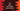# Different ways in C# to print a hollow square pattern## C# program to print a hollow square pattern:

This post will show you how to print a hollow square pattern in C# in different ways. The program will take the size of the pattern as input from the user and print it using star, number or any other character.

We can use any loop to print this pattern. A hollow square pattern of size 6 will look as like below:

``````* * * * * *
*         *
*         *
*         *
*         *
* * * * * *``````

### Algorithm to follow:

The programs will follow the following algorithm:

• Take the size as input from the user. Store it in a variable.
• Run two loops. The outer loop will run for size number of times and the inner loop will also run for size number of times. The outer loop will be used to point to the rows of the pattern and the inner loop will be used to print the content. The outer loop is to point rows and the inner loop is to point columns.
• Print character or number for the first and the last row of the pattern. Also, print for the first and the last column. Print a blank space for other positions.
• Print a new line at the end of each row.

### Example 1: C# program to print a hollow square pattern with star and by using for loops:

Let’s write down the program using for loops and star:

``````namespace Program
{
class Program
{
static void Main(string[] args)
{
int Size;

Console.WriteLine("Enter the size of the square: ");

Console.WriteLine();

for (int row = 0; row < Size; row++)
{
for (int column = 0; column < Size; column++)
{
if (row == 0 || column == 0 || row == Size - 1 || column == Size - 1)
{
Console.Write("* ");
}
else
{
Console.Write("  ");
}
}
Console.WriteLine();
}

}
}
}``````

Here,

• Size is the size of the square. The program asks the user to enter the value of the size and save it to this variable.
• Run two for loops. Both of these loops will run for Size number of times.
• Inside the inner loop we are printing the square. If it is first row or first column or last row or last column, it prints * and a blank space. Else, it prints two blank spaces.
• At the end of each iteration of the inner loop, it prints a new line.

If you run this program, it will print output as like below:

``````Enter the size of the square:
5

* * * * *
*       *
*       *
*       *
* * * * *

Enter the size of the square:
6

* * * * * *
*         *
*         *
*         *
*         *
* * * * * * ``````

### Example 2: C# program to print a hollow square pattern with number and by using for loops:

We can also use any number to print the hollow square pattern. Let’s modify the above program to use an user-input number instead of star:

``````namespace Program
{
class Program
{
static void Main(string[] args)
{
int Size, Num;

Console.WriteLine("Enter the size of the square: ");

Console.WriteLine("Enter a number to print the square: ");

Console.WriteLine();

string Separator = new String(' ', Num.ToString().Length);

for (int row = 0; row < Size; row++)
{
for (int column = 0; column < Size; column++)
{
if (row == 0 || column == 0 || row == Size - 1 || column == Size - 1)
{
Console.Write(Num + Separator);
}
else
{
Console.Write(Separator + Separator);
}
}

for (int i = 0; i < Num.ToString().Length; i++)
{
Console.WriteLine();
}

}

}
}
}``````
• It takes the size of the square as input and stores it in the Size variable.
• It also takes the number to print the square as input and stores it in Num.
• The Separator variable holds the space separator. It is dynamic because we need different spaces between the digits for number with different digit count. For example, a square of 7 will look as like below:
``````7 7 7 7 7 7
7         7
7         7
7         7
7         7
7 7 7 7 7 7 ``````

Similarly, square of 17 will look:

``````17  17  17  17  17  17

17                  17

17                  17

17                  17

17                  17

17  17  17  17  17  17``````

And a square of 177:

``````177   177   177   177   177   177

177                           177

177                           177

177                           177

177                           177

177   177   177   177   177   177 ``````

As you can see here, to create a square of different numbers, we need to calculate the blank spaces between each number dynamically.

The blank space between each number is calculated based on the number of digits. Similarly, space between each line is also calculated dynamically.

• We are using two for loops and the numbers are printed similar to the above program.

It can print squares of any size with any number.